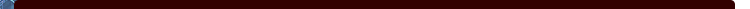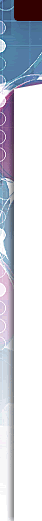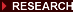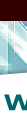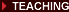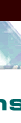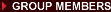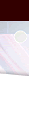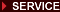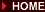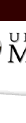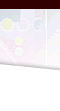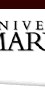Physics 171/171H Introductory Physics: Mechanics and Relativity
Williams course web page, fall 2000

 Old Exams Syllabus Math Review Page Assignment 1 Quiz Solutions Link Deleted Assignment 2 Problem Set Solutions - Link Deleted Assignment 3 Assignment 4 Grade Change Policy Sample Problems Assignment 5 Assignment 6 Exam 1 Solution Link Deleted Assignment 7 Assignment 8 Assignment 9 Assignment 10 Notes from Nov. 2 Exam # 2 Make Up Instructions Assignment 11 Due MONDAY Nov. 20 Exam 2 Solution Link Deleted Problem Set # 12 Exam 3 Solution Link Deleted Assignment #13

Syllabus

M T Th F 9:00 AM, Room 1402 Physics

(171H will meet separately on Thursdays with Prof. Goldenbaum)

Instructor:      Professor E. Williams

Office:      Room 2332 Physics
Phone:     301-405-6156
e-mail:     edw@physics.umd.edu
Study Sessions:     2:30-5:30 Thursday, Conference Room - 2120 Physics

These are informal group study sessions, where the professor or TA will be available to answer questions individually. You should feel free to stop by briefly at any time during the study session to ask a few questions, or to stay there to study or participate in discussions of other students' questions as well as your own.

Course Textbook: Physics, 4th Edition, Volume I, Resnick, Halliday and Krane

Material: Chapters 1-14, 16, 21-25

(not all material in each chapter will be covered)

Reference Texts:    Basic Concepts in Relativity, R. Resnick and D. Halliday Understanding Basic Mechanics, F. Reif

Six Ideas that Shaped Physics: Unit N and Unit C, T. A. Moore

Course Requirements: 1. Homework, 1 set per week, due Fridays at the beginning of class.

2. Attendance and quizzes

3. In Class Exams:   Exam 1:  Tues., Oct. 3
Exam 2:  Mon.,  Oct. 30
Exam 3:  Tuesday, Nov. 21

4. Final Exam: Friday, December 15, 1:30 PM

Grading:       Homework 20%, lowest two sets will be dropped Attendance/Quizzes 5%, will determine borderline grades

In-Class Exams 50% (40% for 171H)

Final Exam 25%

Teaching Assistant (Grader and Study Sessions):    Ng Sheung-Wah
Room 4210 Physics

Miscellaneous Course Information

No late homework will be accepted. Homework sets must show reasoning leading to the final answers in a clear and readable fashion to obtain credit. Multi-page homework sets must be STAPLED. Any loose, corner-folded or paper-clipped homework set will NOT be graded.

Homework assignments will be posted on the course web site. If you didn't get the assignment when announced in class, or if you lost it, you can find it on the site.

Homework, Quiz and Exam solutions will be posted on the course web site only.

Quizzes will be given usually once a week.  The quiz problems will be examples worked in class during the previous lecture, or sample problems from the assigned reading in the text unless otherwise announced.

Assistance outside of the course is available from several sources:

Physics Department Student Services
Provides general academic support for physics majors and students enrolled in physics courses Tom Gleason:  301-405-5979
Bernie Kozlowski:   301-405-5949
Learning Assistance Center   http://www.inform.umd.edu/LASRV
2201 Shoemaker Building,  301-314-7693
Tutoring for Mathematics Courses   http://www.math.umd.edu/undergrad/tutoring.html  Tutoring Service   Anne Arundel Hall - basement (Honors Dept. Office) or
1117 Hornbake Library (Division of Letters and Sciences)
Office of Muti-Ethnic Student Education (OMSE)   http://www.inform.umd.edu/CampusInfo/Departments/OMSE
1101 Hornbake Library Building

Working together on problem sets is strongly encouraged.  However, each student is expected to work through the problem independently, and hand in his or her own work for grading.  For any questions about academic honesty, see  University policies at:

http://www.testudo.umd.edu/soc/dishonesty.html

Quizzes and Exams
If you have a question about grading of a quiz or exam, please see Dr. Williams immediately after the class when the paper was returned.

Homework
The primary purpose of grading the homework is to give you a way of finding out how well you really understand the material.  If you miss points on a problem, that alerts you that you should rework the problem to make sure you understand how to do it properly.  If you feel that you did the problem correctly (and presented your work clearly) and would like to get your homework re-graded, here is the procedure:

1)  Use some common sense.  Any given homework problem is about 1/10 of 1/13 of 1/5 of your grade.  Think about that before spending a lot of time disputing over partial credit.

2)  Don't ask for consideration of changed credit without first looking at the posted solution sets and making sure you really DID do the problem properly.

3)  IF after considering points 1 & 2, you still have a question, then you can write a note explaining the question, attach it, and a copy of the relevant page from the solution set, to your HW set and give it to Dr. Williams, who will forward it to the TA for consideration.  You should circle on your solution set, in a different color ink, the answer that you are worried about.
OR
3')  You can ask the TA for help understanding the problem during the study session.  In this case, please make sure you have a copy of the solution set with you, and have marked the answers you are concerned about on both the solution set and your homework set.

Exam # 2 Make Up Information

For up to 10 extra points added to your Exam # 2 score, you should re-work the problems on the exam - with the changes noted below - taking care to make it VERY EASY AND PLEASANT for the person grading the exam to see what you have done.  The due date is Monday, Nov. 13, in class.  DO NOT hand your original exam back in with corrections marked on it. .  DO NOT hand in pages from your original exam even if your original solution was given full credit.  Partial credit will be awarded FAR LESS liberally than it was in grading the exam, since you have plenty of time to check your work.  No credit will be given unless your work is shown clearly and convincingly.

Changes in working exam:

Repeat problems 1, 3 and 4 as on the original exam.  The corrected figure for problem 3 is: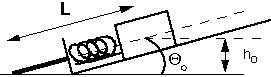(As far as I can tell, everyone who did the problem assumed that this was what I meant.)

Problem 2:  Instead of parts a, b and c, do the following:

Calculate the height mass m2 rises before its speed reaches zero, using:
a)  the work-kinetic energy theorem
b)  Newton's laws (F=ma) directly
(You should get the same answer both ways.)

Remember:
Work-Kinetic Energy Theorem:  The TOTAL work done on a particle is equal to the change in the particle's kinetic energy.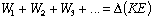If some of the forces doing work are conservative forces where we know the dependence of force on distance, we can calculate a potential energy change and relate its negative to the work done by that force. Then we have: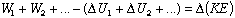This approach is needed for problem 2 and problem 3c.
Remember:
The relationship between period and radius of an orbit was derived FROM the gravitational force law.  If we didn't know the gravitational force law, the period-radius relationship would also be unknown.

listed in reverse chronological order

Suggested study plan:  Begin by reading over the assigned problems for each chapter.  Then look through the figures, tables and sample problems in the chapter.  This will give you an idea of the material is organized and help you focus as you read the chapter.  Then read through the chapter, keeping in mind the questions that have been assigned for homework.  Work through the example problems carefully.  At the end of each section, see if you can do the assigned problems that correspond to that section.  Mark down questions if you get stuck and ask questions in class.  Don't wait until the night before the homework is due to begin working the problems!!  (You'll also get a lot more out of the study sessions if you've looked at the problems before you walk in the door.)

Problem Set # 13

Reading:   Ch. 23, Sections 2,3,4, 6
Ch. 24, Sections 1-4
Problem Set:  Due at the beginning of class, Dec. 12 (note due date is a Tuesday!!)
Supplemental Problems S21, S22, S23
Ch. 23, # 20, 26, 34, 50
Ch. 24 # 6, 14, 22, 24

S.21. A sample of hydrogen gas (hydrogen  = H2, molecular weight = 2.0 grams/mole, distance between H atoms in the molecule is 0.074 nm) is at 500K.  At this temperature, rotations of the molecule are allowed but vibrations are not.
a) What are the average total translational and rotational kinetic energies of a hydrogen molecule?  What is the angular frequency of rotation around one axis?
b) What is the average force (.e. the average over time of many collisions) with which a molecule hits a wall of the container if the walls are 1m apart?
.

S.22 A cubical box with length-of-side L  contains N particles of which
N1 = 1.0 x 1020 have speed 2.0x 102 m/s
N2 = 5.0x1020 have speed 4.5x102 m/s
N3 = 3.x1020 have speed 7.5x102 m/s
The particles are moving randomly within the box and rebound elastically in collisions with each other or the walls.
a) What are the average and rms speeds of the particles
b) What is the temperature of the gas of particles if all the particles have mass 75x10-27 kg?
c) If the molecular diameter of the particles is 2.5x10-10m, find the mean free path in the gas.  Use the average speed for your calculation.

S23. A diatomic ideal gas is held in a box of volume 9 m3, at a temperature
of 500K and a pressure of 0.1 atm. The atoms in the molecules can rotate,
but not vibrate at this temperature.

a) How much gas is in the box?

b) The gas is heated to 600K without changing volume. How much heat
was transferred to the gas?

c) Then the gas is slowly allowed to expand at constant temperature
(600K) until it reaches its original pressure. What is the final volume of
the gas? How much work is done by the gas during this expansion

d) Draw a pressure-volume diagram for the two step expansion of the gas
described in parts b and c. On the same diagram, draw the
pressure-volume line for a one step heat transfer from 500K to 600K at
constant pressure. Explain the difference in the work available from the
two processes.

S24. A monoatomic ideal gas is confined in a two-dimensional container
(only x and y motion allowed) and equilibrated at a temperature of 350K.

a) What is its internal energy? (Hint: how many translational degrees of
freedom are there ?)

b) If the atomic mass of the atoms in the gas is 28.1 g/mole, what is the
root mean square velocity of an atom?

c) The distribution of speeds of the atoms is found to obey the equation:
N(v) = (2pAv)exp{-mv2/2kT)
Find an expression for A in terms of the macroscopic parameters of the
ideal gas.

Problem Set # 12

Ch. 22, Sections 3, 5
Ch. 23, Sections 1, 5
Ch. 25, Sections 1-4
Problem Set:  Due at the beginning of class, MONDAY Dec. 4
Ch. 17, #4, 12
Ch. 22, # 2, 10
Ch. 23, # 2, 4, 36, 40
Ch. 25 # 2, 12, 26
S16

Extra Credit Problems (up to 5 points each on homework score)
S17, S18

S.16    Two skaters, each of mass 50.0 kg, approach each other along parallel pathes separated by 3.0 m.  One skater has velocity 10 m/s and the other has velocity -10 m/s both in the x direction.  The first skater carries a massless pole of length L = 3.0 m.  The second skater grabs the end of it as he passes by.  Assume frictionless ice and treat the skaters as point particles.

a)  What is the velocity of the system center of mass (system = 2 skaters + pole)?

b)  What are the translational and angular velocities of each of the skaters after they are connected by the pole?

c)    By pulling on the pole the skaters reduce their separation to 1.0 m.  What are their translational and angular velocities then?

d)    Compare the kinetic energies of the skaters before the collision, after the collision, and after they have reduced their separation.  Where do the changes come from?

e)    What quantities are conserved in parts b-d?

S.17    Do problem 21 in Chapter 13 of the book.
a)    What is the difference in the mass for d = 0 and for d = L/2?
b)    What is the ratio of rotational to translational kinetic energy after the collision for d = L/4 and for d = L/2?

S.18  Consider the same configuration as for problem S.17 (a point particle hitting a thin rod normal to its length), but now the collision is inelastic.  Compare the change in kinetic energy during the collision for d = 0 and for d = L/2.  Use the values  L = 2.2 m, m1 (the point particle ) = 2.0 kg, m2 (the rod) = 2.5 kg, and vo (the initial speed of the point particle) = 1 m/s.

Problem Set # 11

Reading:   Ch. 12 Sections 1-6, Ch. 13 Sections 1-4
Problem Set:   Due at the beginning of class, MONDAY, Nov. 20
Ch. 12, # 6, 8, 20, 24, 44, 50
Ch. 13, #2, 12, 28, 34, 36
Problem S13
Extra Credit (up to 20% extra on this hw score) S14, S15

If you are having trouble with concepts or problem solving in chapter 10, you might find it helpful to see a different approach in the supplemental material:

Circular motion, rotational motion, angular momentum:  Reif Chs. 8, 17, 18
Rotational Energy and Angular Momentum:  Moore, Volume C, Chs. 9 and 13

S13  A particle of mass m is attached to a string which passes through a hole in a table.  The particle rotates without friction on the table around the hole with speed v and radius R.
a) What is the tension in the string?
b) If one pulls on the string until the mass moves inward to 1/4 the original radius, what is the new speed of rotation?  What is the new tension in the string?
c)   Show two different ways to determine the amount of work done in moving the mass inward, and confirm that the two approaches give the same answer.

S14  A small mass, m, is moving in the vicinity of a large mass M.  The large mass is so much larger that it undergoes no observable acceleration due to its gravitational interaction with the small mass.   (Bold symbols, for instance r2, indicate vectors.  Non-bold symbols, for instance r2, indicate the magnitude of the vector.)  The zero of the coordinate system is the center of the large mass.
a) The small mass is observed at time t1 to be at a location r1  and traveling with speed v1 .  At a later time, t2 , it is at a location r2 .  The magnitude of r2   is r2  = 0.1r1 .  What is the speed of the particle at the later time?  Express your answer in terms of G, M, r1  and v1 .
b) The velocity at time t2  is observed to be perpendicular to the location vector  r2 .  What is the angle between the location vector and the velocity vector at time  t1 ?  Draw a diagram indicating clearly the angle you have determined.

S15 A large solid sphere of mass m2 = 5.0 kg and radius R = 0.50 m is suspended from a pivot by a rigid massless rod.  The distance of the center of mass of the sphere from the pivot is   L = 1.2 m.  A point particle of mass m1 =2.5 kg and a horizontal initial velocity of v0 = 6.0 m/s hits the large sphere head-on and sticks to it.
a) What is the angular velocity of the combined masses just after the collision?
b) Assume the collision lasts 0.0020s, what is the average force on m1 during the collision?  What is the torque exerted on m1?
c) Show that the final angular velocity of m1 is consistent with the torque exerted on it.

Problem Set # 10

Reading: Ch. 10, Sections 1-5, Ch. 11, Ch. 21, Section 8

If you are having trouble with concepts or problem solving in chapter 10, you might find it helpful to see a different approach in the supplemental material:

Collisions:  Reif Ch. 16, Section C
Collisions and Center of Mass:  Moore, Volume C, Sections 4.5 5.5, 12.7

Problem Set:  Due at beginning of Class, Nov. 10
Chapter 10, # 8, 10, 26, 28, 32, 46
Chapter 11, #26, 32
Suppl. Problem S10, S11
Extra Credit (up to 20% extra on this hw score) S 12

S10. A stationary nucleus of mass 107mp, where mp is the rest mass of a proton, breaks apart (decays) into two particles.  One of the particles has rest mass m1 = 20mp and speed v1 = 0.89c.
a) Find the rest mass m2 and speed v2 of the other particle.  Express your answers in terms of mp and c.
b) Kinetic energy has increased in this decay.  Explain why.

S11. If a relativistic collision occurs elastically, that is with no change in the total kinetic energy of the particles involved, what is the change in the total mass of the particles involved?

EXTRA CREDIT:
S12.  In an isolated system (no external forces) of two particles, mass m1 is moving at velocity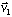and
mass m2 is moving at velocity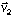.  The vector between the two particles is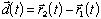a) Find an expression for the center of mass position in terms ofb) Find expressions for the total momentum of this system in:
i)  the given reference frame
ii)  the reference frame in which m1 is initially stationary
iii)  the reference frame in which the COM is stationary.

Problem Set # 9

Problem Set,  Due at beginning of Class, Nov. 3
Chapter 9 # 10, 16, 18, 24, 36, 38

If you are having trouble with concepts or problem solving in chapter 9, you might find it helpful to see a different approach in the supplemental material:

Momentum and Center of Mass:  Reif Ch. 15
Momentum and Center of Mass:  Moore, Volume C, Ch.4 & 5

Problem Set # 8

Reading:  Chapter 8, Sections 1-3, 7; Chapter 16, Section 6; Ch. 21 Section 9
Problem Set,  Due at beginning of Class, Oct. 27
Chapter 8 # 8, 14, 20, 30, 52, 66
Chapter 16 #  30, 36, 40
Chapter 21 #  50, 52

If you are having trouble with concepts or problem solving in chapter 8, you might find it helpful to see a different approach in the supplemental material:

Potential Energy:  Reif Ch. 14
Kinetic and Potential Energy:  Moore, Volume C, Ch.6 & 7

Problem Set # 7

Reading: Chapter 7; Ch. 16, Sections 4 and 8 (through p. 358)
Problem Set,  Due at beginning of Class, Oct. 20
Chapter 7 # 8, 10, 14, 26,  38, 50, 62
Chapter 16, #  44, 46
Supplemental Problem S9

If you are having trouble with concepts or problem solving in chapter 7, you might find it helpful to see a different approach in the supplemental material:

Work and kinetic Energy:  Reif Ch. 13
Problem S9:

An object of mass 0.6 kg is sliding around the inside of a cylinder of radius 1.8 m.  One half of the cylinders inner surface is frictionless, and the other half is rough with kinetic coefficient of friction mk = 0.2.  The mass initially is moving on the smooth surface with constant speed of 2 m/s.

a)   Draw a force diagram for the object after it has entered the rough part of the cylinder.  What is the magnitude of the frictional force just after the object has entered the rough part?

b) What is the velocity of the particle one second after it has entered the rough part of the cylinder?  (Use Dv = aDt).

Problem Set # 6

Reading: Chapter 6, Sections 1-5, Chapter 16, Sections 1-4
Problem Set,  Due at beginning of Class, Oct. 13
Chapter 6, # 10, 16, 24, 28, 34, 36, 40, 50
Chapter 16, # 6, 12

If you are having trouble with concepts or problem solving in chapter 6, you might find it helpful to see a different approach in the supplemental material:

Springs, Friction, Orbits:  Reif Ch. 8, 12
Constrained Motion:  Moore, N7-9

Problem Set # 5

Problem Set,  Due at beginning of Class, Oct. 6
Chapter 5,  # 4, 10, 18, 36, 38, 46, 50, 56

If you are having trouble with concepts or problem solving in chapter 5, you might find it helpful to see a different approach in the supplemental material:

Newtonian Mechanics:  Reif Ch. 9-11
Force Diagrams:  Moore, N3.7, 4.3, 4.4, 4.8

Assignment Set # 4

Reading: Chapter 21, Sections 3 - 7
Problem Set,  Due at beginning of Class, Sept. 29
Chapter 21 # 2, 4, 14, 16, 20, 22
Supplemental Problems S5, S6, S7, S8

S5. Among the particles of high-energy physics are charged pions.  They can be produced by bombarding a target in an accelerator with high-energy protons.  The pions leave the target with speed close to that of light.  The pions are radioactive, and when they are brought to rest, their half-life is measured to be 1.8x10-8 s.  That is half of the number present at any time have decayed 1.8 x 10-8s  later.  In a collimated pion beam, of speed 0.99c, 50.0% of the pions have decayed by the time the beam has traveled to 38m from the target.
a) Are these results consistent if we ignore the effects of relativity?  (Show  reasoning.)
b) Show how the time dilation accounts for the measurements.
c) Show how the length contraction accounts for the measurements.

S6. Two spaceships, each of proper length 100m, pass near one another heading in opposite directions.  An astronaut at the front of one ship (S) measures a time interval of 2.5x10-6 s for the second ship (S) to pass.
a)   What is the relative speed v of the two ships?
b)   What time interval is measured on ship S for the front of ship S to pass from the front to the back of S?

S7. Observer S reports that an event occurred on his x axis at x = 3.0x108 m at a time t = 2.50s.
a) Observer S is moving in the direction of increasing x at a speed of 0.40c.  What coordinates would he report for the event?
b) What coordinates would Observer S report if he were moving in the direction of decreasing x at this same speed?

S8. A clock moves along the x axis at a speed of 0.60c and reads zero as it passes the origin.
a) Use a Lorentz transform to determine the time it reads as it passes the 180m mark on this axis.
b) Explain why you can or cannot do this problem using the time dilation formula.

Assignment Set # 3

Reading: Chapter 4, Sections 4-6, Chapter 21, Sections 1-3 and 5
Problem Set,  Due at beginning of Class, Sept. 22
Chapter 4  # 52, 56, 72, 76, 78
Chapter 21  # 2, 4,
Supplemental Problems  S1, S2, S3, S4 (below, or download .pdf file )

If you are having trouble with concepts or problem solving , you might find it helpful to see a different approach in the supplemental material:

Circular motion and relative motion:  Reif, Ch. 8; Moore Unit N, Ch. 9 and 10

S1. Vector description of circular motion.
Suppose we describe the position of a particle with a vector R = RcosQ i + RsinQ j, where r is the distance of the particle from the origin of the reference frame, Q is the angle measured counterclockwise from the positive x axis, and  i and  j are the unit vectors in the x and y directions respectively.  If the particle is moving in a circle with constant speed, the value of R remains constant, and the angle Q changes linearly with time according to Q = at.  Given a = 360o  per second, and R = 2m, make three plots:

Plot the x position of the particle vs. time
Plot the y position of the particle vs. time
Plot the trajectory of the particle (its path in the xy plane), labeling the positions corresponding to Q = 45o, 180o and 315o with the time at which each of those positions is reached.  Draw arrows indicating the directions of the velocity vector and the acceleration vector at each of those three points as well.
S2.   A firecracker explodes 30 km away from an observer, who is sitting next to a clock A.  The light from the firecracker explosion reaches the observer at exactly t = 0, according to clock A.  Imagine that the flash of the explosion illuminates the face of another clock B which is sitting next to the firecracker.  What time would clock B register at the moment of illumination, if it is correctly synchronized with clock A?  Express your answer in milliseconds.

S3.   Imagine that you are in an inertial frame in empty space with a clock, a telescope and a powerful strobe light.  A friend is sitting in the same frame a very large (unknown) distance from your clock.  At precisely 12:00:00 noon, according to your clock, you set off the strobe lamp.  Precisely 30.0 seconds later, you see in your telescope the flash of your friends clock illuminated by your strobe flash.  How far away is your friend?  What should you see on the face of your friends clock if that clock is synchronized with yours?

S4.    Physical understanding of trajectories: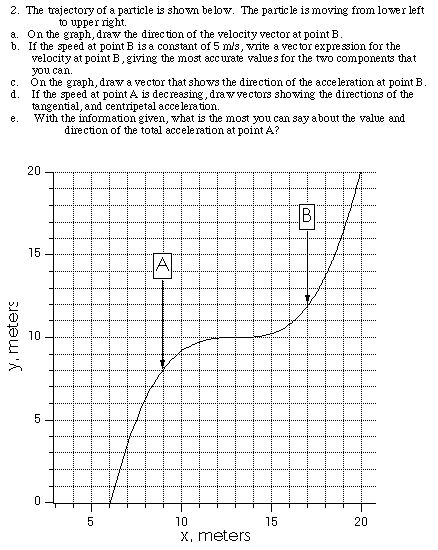Assignment Set # 2

Reading: Chapter 3, Sections 1-5, Chapter 4, Sections 1-3
Problem Set,  Due at beginning of Class, Sept. 15
Chapter 3 # 8, 10, 16, 20, 24, 38, 48
Chapter 4 # 8, 14, 24, 40, 44

If you are having trouble with concepts or problem solving in chapters 3 and 4, you might find it helpful to see a different approach in the supplemental material:

Trig, vectors:  Moore (Six Ideas, Unit C), p. 38, 54, 179-80
Calculus: Moore (Six Ideas, Unit N), p. 10, 54, 69-70
Projectile Motion:  Reif, Ch. 7;  Moore, Unit N, Ch. 11

Assignment Set # 1

Problem Set 1, Due at beginning of Class, Sept. 8
Chapter 1  # 18, 30, 40
Chapter 2  # 14, 18, 22, 30, 40, 48, 60, 66

If you are having trouble with concepts or problem solving in chapters 1 and 2, you might find it helpful to see a different approach in the supplemental material:

Graphical analysis of 1-dimensional Motion:  Moore, Unit N, p. 51-52
Problem solving approaches for Motion in a Straight Line:  Reif, Chapters 4-5

top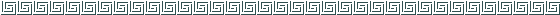Syllabus

Physics 171/171H - Spring 2000

Syllabus

Physics 171/171H - Spring 2000